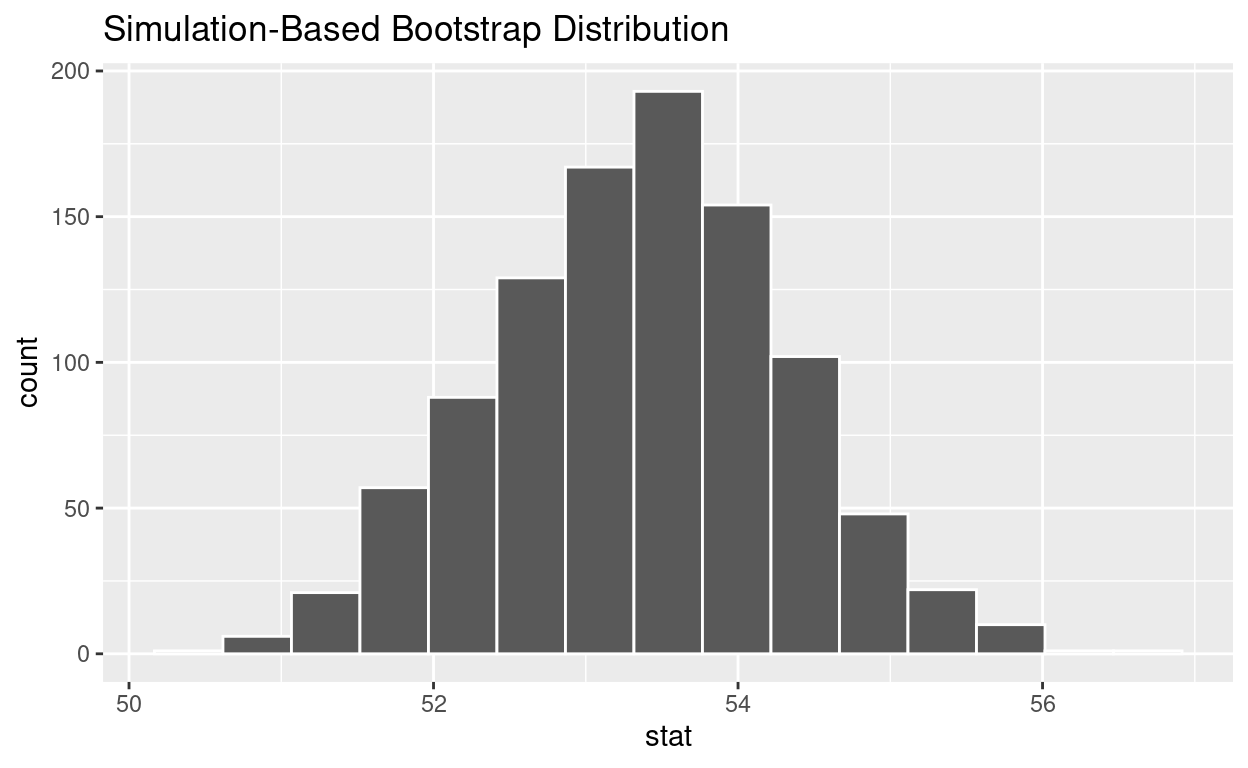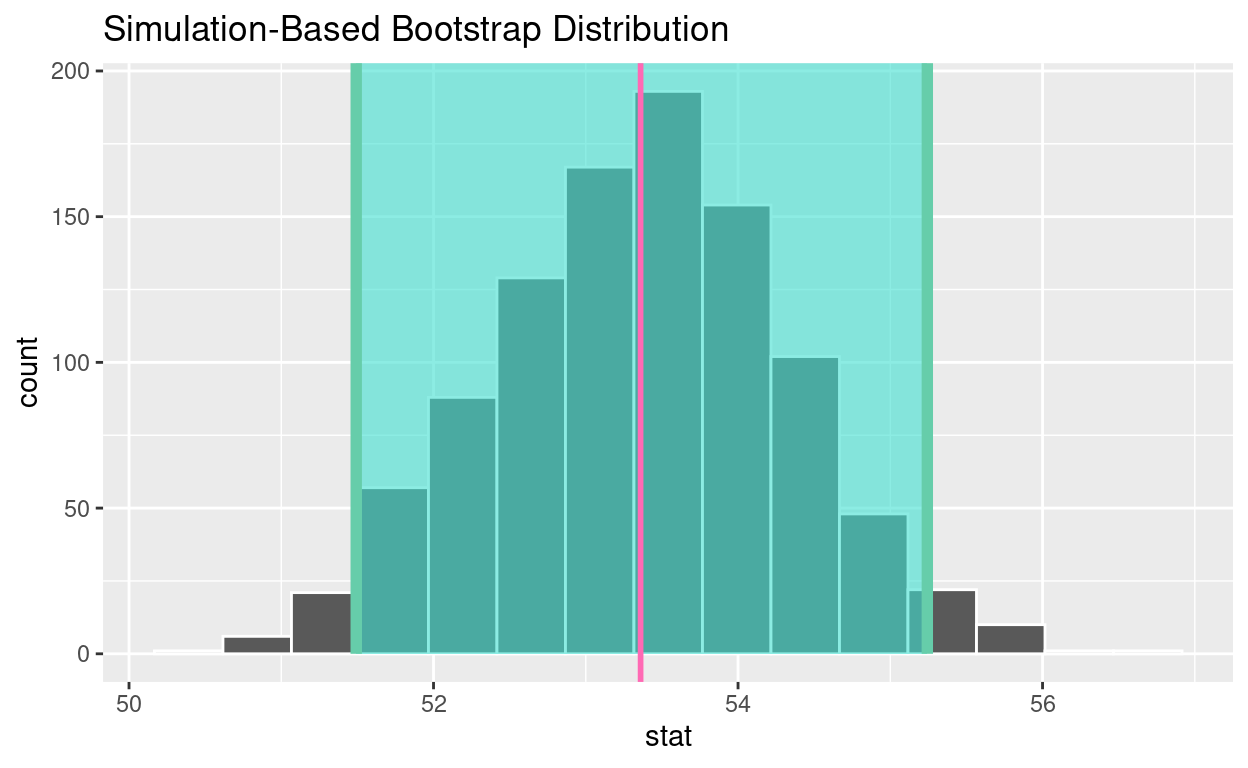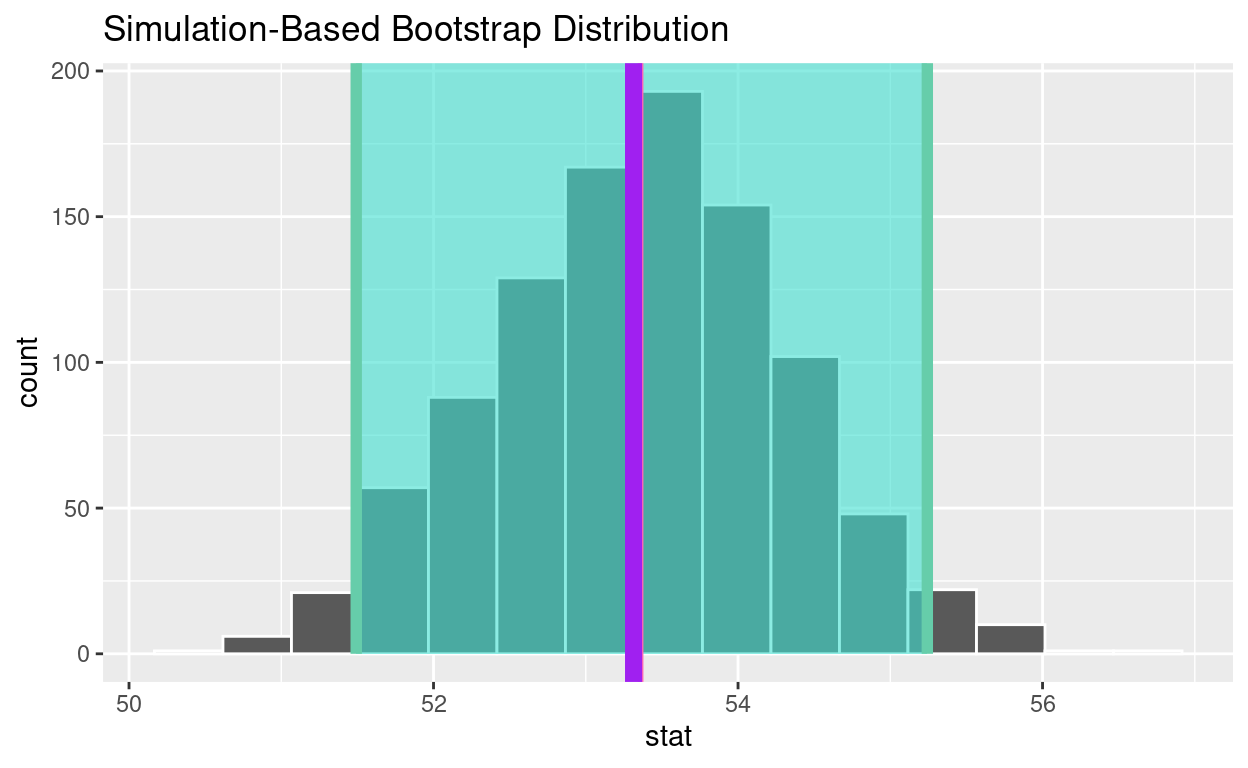# 10: Bootstrapping and Confidence Intervals

Based on Chapter 8 of Modern Dive. Code for Quiz 12

Load the R packages we will use

``````library(tidyverse)
library(moderndive)
library(infer)
library(fivethirtyeight)
``````
• Replace all the instances of ???. These are answers on your moodle quiz.

• Run all the individual code chunks to make sure the answers in this file correspond with your quiz answers

• After you check all your code chunks run then you can knit it. It won’t knit until the ??? are replaced

• Save a plot to be your preview plot`

• Look at the variable definitions in `congress_age`

What is the average age of members that have served in congress?

• Set random seed generator to 123

• Take a sample of 100 from the dataset `congress_age` and assign it to `congress_age_100`

``````set.seed(123)

congress_age_100 <- congress_age  %>%
rep_sample_n(size=100)
``````
• congress_age is the population and congress_age_100 is the sample

• 18,635 is number of observations in the population and 100 is the number of observations in your sample

Construct the confidence interval

1. Use `specify` to indicate the variable from congress_age_100 that you are interested in
``````congress_age_100  %>%
specify(response = age)
``````
``````Response: age (numeric)
# A tibble: 100 × 1
age
<dbl>
1  53.1
2  54.9
3  65.3
4  60.1
5  43.8
6  57.9
7  55.3
8  46
9  42.1
10  37
# … with 90 more rows``````

2. `generate` 1000 replicates of your sample of 100

``````congress_age_100  %>%
specify(response = age)  %>%
generate(reps = 1000, type= "bootstrap")
``````
``````Response: age (numeric)
# A tibble: 100,000 × 2
# Groups:   replicate [1,000]
replicate   age
<int> <dbl>
1         1  42.1
2         1  71.2
3         1  45.6
4         1  39.6
5         1  56.8
6         1  71.6
7         1  60.5
8         1  56.4
9         1  43.3
10         1  53.1
# … with 99,990 more rows``````

3. `calculate` the mean for each replicate

• Assign to `bootstrap_distribution_mean_age`

• Display `bootstrap_distribution_mean_age`

``````bootstrap_distribution_mean_age  <- congress_age_100  %>%
specify(response = age)  %>%
generate(reps = 1000, type = "bootstrap")  %>%
calculate(stat = "mean")
``````
• The bootstrap_distribution_mean_age has 1000 means

4. `visualize` the bootstrap distribution

``````visualize(bootstrap_distribution_mean_age)
``````Calculate the 95% confidence interval using the percentile method

• Assign the output to `congress_ci_percentile`

• Display `congress_ci_percentile`

``````congress_ci_percentile  <- bootstrap_distribution_mean_age %>%
get_confidence_interval(type = "percentile", level = 0.95)

congress_ci_percentile
``````
``````# A tibble: 1 × 2
lower_ci upper_ci
<dbl>    <dbl>
1     51.5     55.2``````

Calculate the observed point estimate of the mean and assign it to `obs_mean_age`

• Display `obs_mean_age`
``````obs_mean_age  <-  congress_age_100  %>%
specify(response = age)  %>%
calculate(stat = "mean")  %>%
pull()
``````

• Add a line at the observed mean, `obs_mean_age`, to your visualization and color it “hotpink”

``````visualize(bootstrap_distribution_mean_age) +
geom_vline(xintercept = obs_mean_age, color = "hotpink", size = 1 )
``````• Calculate the population mean to see if it is in the 95% confidence interval

• Assign the output to `pop_mean_age`

• Display `pop_mean_age`

``````pop_mean_age  <- congress_age  %>%
summarize(pop_mean= mean(age))  %>% pull()

pop_mean_age
``````
`` 53.31373``
• Add a line to the visualiztin at the, population mean, `pop_mean_age`, to the plot color it “purple”
``````visualize(bootstrap_distribution_mean_age) +
geom_vline(xintercept = obs_mean_age, color = "hotpink", size = 1) +
geom_vline(xintercept = pop_mean_age, color = "purple", size = 3)
````````````ggsave(filename = "preview.png",
path = here::here("_posts", "2022-04-25-10-bootstrapping-and-confidence-intervals"))
``````
• Save the previous plot to preview.png and add to the yaml chunk at the top

• Is population mean the 95% confidence interval constructed using the bootstrap distribution? yes

• Change set.seed(123) to set.seed(4346). Rerun all the code.

• When you change the seed is the population mean in the 95% confidence interval constructed using the bootstrap distribution? no

• If you construct 100 95% confidence intervals approximately how many do you expect will contain the population mean? 95